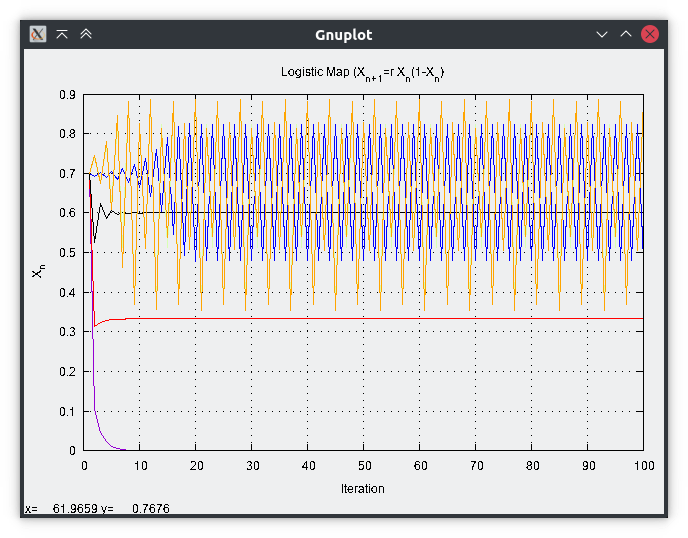# 100 Days of Code Log R1D4

Today, I was introduced to I/O in Fortran. Its a little complex for seemingly simple task. But, its good to have all the bells and whistles known upfront. Here is a sample program on input output.

 `````` 1 2 3 4 5 6 7 8 9 10 11 12 13 14 15 16 17 18 19 20 21 22 23 24 `````` ``````program inputoutput use, intrinsic :: iso_fortran_env, only : sp=>real32, dp=>real64, error_unit, input_unit implicit none integer :: unit_nr, istat integer :: a, b character(len=1024) :: msg ! IO Opening a file open(newunit=unit_nr, file='text.txt', access='sequential', action='write', & status='new', form='formatted', iostat=istat, iomsg=msg) if (istat /= 0 ) then ! Handling Error write (unit=error_unit, fmt='(3A)') 'error: ', trim(msg), istat stop 1 end if ! Writing to a file write (unit=unit_nr, fmt='(A)') 'hello world' write (unit=unit_nr, fmt='(F4.2)') x close(unit=unit_nr) print *, "Enter the number" ! Reading input from user read(unit=input_unit, fmt='(I2)', iostat=istat, iomsg=msg) a print *, a+5 end program inputoutput ``````

In Fortran IO, there are few points to note.

• Each file is represented by a unit number. While opening a file, it can either be specified manually (`open(unit=unit_number)`) or assigned at the time of opening with `newunit` keyword.
• Access can be `sequential` `direct` or `stream`.
• action can be `read`, `write` or `readwrite`.
• status - `old`, `new`, `replace` or `scratch`.
• form - `formatted` or `unformatted`.
• posiition (for writing) - `rewind` or `append`.
• iostat - the integer which will contain the exit status of command. If the variable is not equal to zero, the error handling has to be performed.
• iomsg - a string which will carry the error message needs to be passed.

Reading the input follows a similar suite, though for reading from user console, the parameter is `unit=input_unit` which is available in `iso_fortran_env`.

I still haven’t understood how the format is specified but have left it for future. I have started with the exercises but will post more on that on the upcoming day! Here is a logistic map from the first exercise!